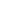# Problem: What is the standard cell potention, E°, for this half-reaction? Pd2+(aq) + 2e– → Pd(s) Standard Reduction Potentials                              E°        Cu(s) + Pd 2+ → Cu 2+(aq)  + Pd(s)              + 0.650 V Cu 2+ (aq) + 2e– → Cu(s)                               + 0.337 V   A) + 0.987 V B) –0.987 V C) + 0.313 V D) – 0.313 V

###### FREE Expert Solution
95% (154 ratings)
###### Problem Details

What is the standard cell potention, E°, for this half-reaction?

Pd2+(aq) + 2e → Pd(s)

Standard Reduction Potentials                              E°

Cu(s) + Pd 2+ → Cu 2+(aq)  + Pd(s)              + 0.650 V

Cu 2+ (aq) + 2e– → Cu(s)                               + 0.337 V

A) + 0.987 V

B) –0.987 V

C) + 0.313 V

D) – 0.313 VWhat scientific concept do you need to know in order to solve this problem?

Our tutors have indicated that to solve this problem you will need to apply the Cell Potential concept. If you need more Cell Potential practice, you can also practice Cell Potential practice problems.

What is the difficulty of this problem?

Our tutors rated the difficulty ofWhat is the standard cell potention, E°, for this half-react...as low difficulty.

How long does this problem take to solve?

Our expert Chemistry tutor, Jules took 1 minute and 59 seconds to solve this problem. You can follow their steps in the video explanation above.

What professor is this problem relevant for?

Based on our data, we think this problem is relevant for Professor Bindell's class at UCF.